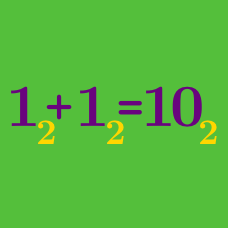Number Theory

# Alternate Bases

Express the base 4 number $123_{4}$ as a decimal number.

Convert the decimal number 96 to base 5.

If $n$ is a positive natural number, how many $1$'s will appear in the binary (base 2) representation of

$2^n + 1\text{?}$

What is the next natural number after $33_4\text{?}$

Which of the following is the binary (base 2) representation of $\frac{7}{8}$?

×

Problem Loading...

Note Loading...

Set Loading...